# ALPHY5: WAVES

##### This unit explains waves in Senior Five

Basic Properties Of Waves

A wave can be defined as the transfer of energy between two points without any physical transfer of matter. Waves on the surface of the sea or on a lake provide an obvious example, because they are highly visible. The fact that they transfer energy can be seen from the effects of coastal erosion over many years, and from the more immediate effects involving the transfer of materials onto the shoreline. Sound is an example of waves that we can hear, and is caused by vibrating air molecules.

Amplitude.

Amplitude is a measure of how big the wave is.It measured as, the height from the equilibrium point to the highest point of a crest or the depth from the equilibrium point to the lowest point of a trough.Wave Length.

Wavelength is an important property of a wave which is illustrated in the figure. We know that a wave is a repeating pattern. It repeats itself in a periodic manner and a regular way over both space and time. This  wavelength can be calculated as the distance from trough to trough or from crest to crest. Wavelengths represent a repeating pattern of traveling energy, such as light or sound. Their distinctive formations play an important role in distinguishing one type of energy from another.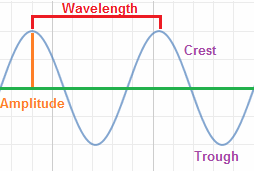### Wavelength formula

A wavelength can be calculated by dividing the velocity of a wave by its frequency. This is often expressed as the following equation:

The Greek letter lambda (λ) represents wavelength, expressed in meters. The vis wave velocity, calculated as meters per second. And the f stands for frequency, which is measured in hertz.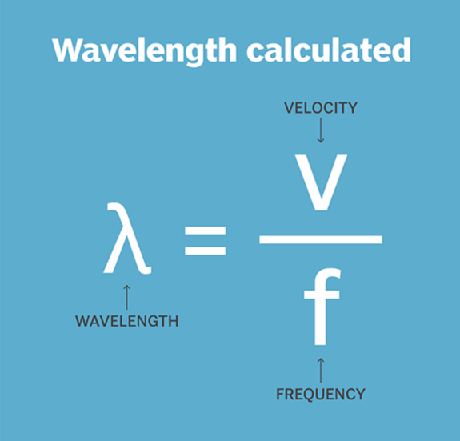Wave PeriodA typical wave motion is described in the above diagram. The motion is not along a straight line but it is the form shown by the red curve. Consider a particle at the mean position initially. In the direction of motion of the wave, with the passage of time, the particle starts rising till it reaches a peak called crest, then starts falling till it reaches the lowest point crossing the mean position. The lowest point is called trough.Again it starts rising and reaches the mean position. In other words the particle has completed one cycle of wave motion. This cycle repeats in a uniform pattern along with time.

Since the wave motion is uniform, the time taken for a particle to complete one cycle is a constant for the particular wave motion and it is defined as the period of the wave. It is generally represented by the letter T.
Thus we can define time period as:

The length that a particle has traveled in one period of a wave is called wave length of the wave motion and denoted by the Greek letter λ

λ(pronounced as lambda).
Now let us say that the particle completes ‘f’ cycles in a second. Therefore, we can write an equation as,

×

× T = 1. The letter f which represents the number of cycles per second is defined as the frequency of the wave motion. Thus the frequency and the period of wave are reciprocals to each other.

Formula For Period Of A Wave

Let us set up an equation for a wave motion. Let us refer to the same diagram and let us assume that the velocity of the wave as ‘v’. Hence, in one second the wave moves by a distance ‘v’ units. Therefore in the time period of a wave T, the wave moves by a distance of V ×

× T units. But on the other hand, we defined the same distance as the wave length λ

λ.
therefore

therefore V ×

× T = λ

λ or

V = λT

λTor

V = f λ

λ

Period Of A Sin Wave

The wave motions of several phenomenons are sine waves or sinusoidal waves. That is when an object is rotating around a point, the height of the object at any time is the sine function of the angle made by the object with positive x-axis. That is the displacement of a particle in this type of wave motion is a sine function of time. The basic form of a sine function is,

y = sin (ω

ω t),

where ω

ω is the angular frequency and
y is the displacement at any time ‘t’.
For example, the height of a passenger in a Ferris wheel with respect to the horizontal axis of the wheel is an sine wave motion.
We know that a sine function completes an angle of 2 π

π radians in one cycle. Now the time taken for completing 2 π

π radians calculated from the angular velocity ω

ω. As per the definition of angular velocity ω

ω, the time required for an angle of 2 π

2πω second. In other words, for completing one cycle of sine wave motion the time required for one cycle is 2πω

2πω, which is nothing but T, the period of a wave.

∴ the formula for the Sine Wave Period is derived as

T = 2πω

2πω.

We can derive the same formula by another method as explained below. In one revolution the angle covered is 2Π

Π radians. Suppose the frequency of wave motion is ‘f’, then in one second the angle covered is 2Pi

Pif radians, which is, same as the angular frequency ω

ω. Therefore,
π

π f = ω

ω or,

f = ω2π

Frequency

Frequency (ƒ) is the term used to describe the number of oscillations (cycles) per second of a wave. The unit of frequency is the Hertz (Hz), and one Hertz is equal to one cycle per second. The term is named after the German physicist Heinrich Rudolph Hertz, who first produced and observed electromagnetic waves in 1887. The term is combined with metric prefixes to denote multiple units such as the kilohertz (103 Hz), megahertz (106 Hz), and gigahertz (109 Hz).  Frequency (f), can be obtained by dividing the wave’s velocity, usually symbolized by the letter v, by its wavelength. Remember, we represent it with the Greek symbol: lambda. We usually measure the wavelength in meters and the velocity in meters per second. The frequency found using these units will be measured in Hz (hertz), another way of saying cycles per second.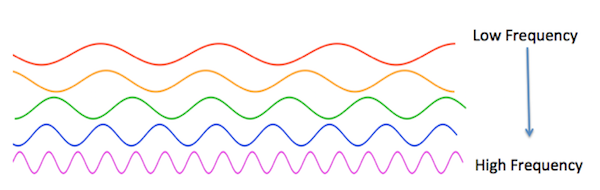Speed

A wave is nothing but the disturbance which is moves along the medium from one end to another. The speed is a property of wave which refers how fast the object moves through a medium. In the case of a wave, the speed is the distance traveled by a given point on the wave in a given interval of time.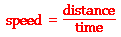Wave Theory

The wave theory of light, proposed by Dutch physicist Christian Huygens in 1678, has stood the test of time and is today considered one of the backbones of optics.

Definition of Light

Before going into the wave theory of light, let’s learn about light itself. You may be aware by now that light is a form of energy. It is the only form of energy that is visible to the naked eye. In more precise terms, light is a part of the electromagnetic spectrum, which includes visible light, radio waves (AM, FM and SW), microwaves, x-rays and gamma rays.

Background to the Wave Theory of Light

Light has always intrigued scientists and thinkers. But it was not until the late 17th century that scientists began to study its properties. Sir Isaac Newton thought that light was made of tiny particles. But Dutch physicist Christian Huygens believed that it was made of waves that propagated by vibrating up and down perpendicular to the direction of its movement.

In 1678, Huygens proposed that every point that a luminous disturbance meets turns into a source of the spherical wave itself. The sum of the secondary waves, which are the result of the disturbance, determines what form the new wave will take. This theory of light became known as ‘Huygens’ Principle’.

Using this principle, Huygens was successful in deriving the laws of reflection and refraction of light. He was also successful in providing an explanation for the linear and spherical propagation of the light waves. However, he was not able to explain the diffraction effects. As you may already know, diffraction effects are the bending of light waves that take place when they hit the edges of an obstacle or opening, screens and apertures. The explanation was provided by French physicist Augustin-Jean Fresnel in 1816.

Huygens’ Principle was proved to be correct by Thomas Young in 1803. While studying the interference of light, he showed that when light is shone through a screen with two slits that are equally separated, the light emerging from them spread out according to Huygens’ Principle. In 1815, Fresnel provided mathematical equations for Young’s experiment.

In 1900, physicist Max Planck proposed that light was made of finite packets of energy, called a light quantum, which depends on the velocity and frequency of light. In 1905, Einstein proposed that light had the characteristics to support both particle and wave theory. Based on Planck’s work on the emission of light from hot bodies, he suggested that light is made of tiny particles called photons.

Later, quantum mechanics gave proof of the dual nature of light.

Huygens’ Principle

Huygens’ Principle (also called Huygens-Fresnel Principle) states that every point on a wave front is a source of wavelets that spread forward at the same speed. It can be shown mathematically by the following equation:

s = v x t

where s is the distance travelled, v is the speed of propagation and t is the time.

Each point on a wave front emits semi-circular waves at speed v. The waves which occur after some time, t. These waves create a new wave front, which is tangent to the wavelets.

This principle works for all types of waves, including sound waves, and is helpful in describing the other phenomena of light, namely reflection, refraction and interference.

Interference Of Waves

Wave interference is the phenomenon that occurs when two waves meet while traveling along the same medium. The interference of waves causes the medium to take on a shape that results from the net effect of the two individual waves upon the particles of the medium. To begin our exploration of wave interference, consider two pulses of the same amplitude traveling in different directions along the same medium. Let’s suppose that each displaced upward 1 unit at its crest and has the shape of a sine wave. As the sine pulses move towards each other, there will eventually be a moment in time when they are completely overlapped. At that moment, the resulting shape of the medium would be an upward displaced sine pulse with an amplitude of 2 units. The diagrams below depict the before and during interference snapshots of the medium for two such pulses. The individual sine pulses are drawn in red and blue and the resulting displacement of the medium is drawn in green.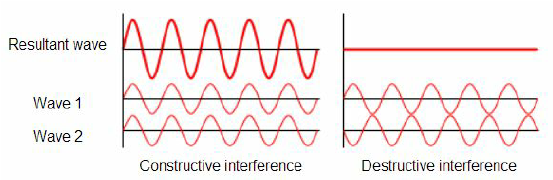### Constructive Interference

This type of interference is sometimes called constructive interference. Constructive interference is a type of interference that occurs at any location along the medium where the two interfering waves have a displacement in the same direction. In this case, both waves have an upward displacement; consequently, the medium has an upward displacement that is greater than the displacement of the two interfering pulses. Constructive interference is observed at any location where the two interfering waves are displaced upward. But it is also observed when both interfering waves are displaced downward. This is shown in the diagram below for two downward displaced pulses.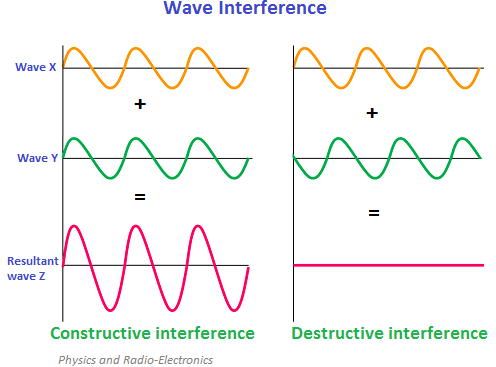### Destructive Interference

Destructive interference is a type of interference that occurs at any location along the medium where the two interfering waves have a displacement in the opposite direction. For instance, when a sine pulse with a maximum displacement of +1 unit meets a sine pulse with a maximum displacement of -1 unit, destructive interference occurs.

The result is that the two pulses completely destroy each other when they are completely overlapped. At the instant of complete overlap, there is no resulting displacement of the particles of the medium. This “destruction” is not a permanent condition. In fact, to say that the two waves destroy each other can be partially misleading. When it is said that the two pulses destroy each other, what is meant is that when overlapped, the effect of one of the pulses on the displacement of a given particle of the medium is destroyed or canceled by the effect of the other pulse.

Diffraction Of Waves

Reflection involves a change in direction of waves when they bounce off a barrier; refraction of waves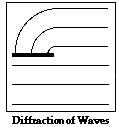involves a change in the direction of waves as they pass from one medium to another; and diffraction involves a change in direction of waves as they pass through an opening or around a barrier in their path. Water waves have the ability to travel around corners, around obstacles and through openings. This ability is most obvious for water waves with longer wavelengths. Diffraction can be demonstrated by placing small barriers and obstacles in a ripple tank and observing the path of the water waves as they encounter the obstacles. The waves are seen to pass around the barrier into the regions behind it; subsequently the water behind the barrier is disturbed. The amount of diffraction (the sharpness of the bending) increases with increasing wavelength and decreases with decreasing wavelength. In fact, when the wavelength of the waves is smaller than the obstacle, no noticeable diffraction occurs.

Diffraction of water waves is observed in a harbor as waves bend around small boats and are found to disturb the water behind them. The same waves however are unable to diffract around larger boats since their wavelength is smaller than the boat. Diffraction of sound waves is commonly observed; we notice sound diffracting around corners, allowing us to hear others who are speaking to us from adjacent rooms. Many forest-dwelling birds take advantage of the diffractive ability of long-wavelength sound waves. Owls for instance are able to communicate across long distances due to the fact that their long-wavelength hoots are able to diffract around forest trees and carry farther than the short-wavelength tweets of songbirds. Diffraction is observed of light waves but only when the waves encounter obstacles with extremely small wavelengths (such as particles suspended in our atmosphere)

Stationary Waves

standing wave pattern is a vibrational pattern created within a medium when the vibrational frequency of the source causes reflected waves from one end of the medium to interfere with incident waves from the source. This interference occurs in such a manner that specific points along the medium appear to be standing still. Because the observed wave pattern is characterized by points that appear to be standing still, the pattern is often called a standing wave pattern. Such patterns are only created within the medium at specific frequencies of vibration. These frequencies are known as harmonic frequencies, or merely harmonics. At any frequency other than a harmonic frequency, the interference of reflected and incident waves leads to a resulting disturbance of the medium that is irregular and non-repeating.How A Standing Wave Is Formed.

let’s consider a snakey stretched across the room, approximately 4-meters from end to end. (A “snakey” is a slinky-like device that consists of a large concentration of small-diameter metal coils.) If an upward displaced pulse is introduced at the left end of the snakey, it will travel rightward across the snakey until it reaches the fixed end on the right side of the snakey. Upon reaching the fixed end, the single pulse will reflect and undergo inversion. That is, the upward displaced pulse will become a downward displaced pulse. Now suppose that a second upward displaced pulse is introduced into the snakey at the precise moment that the first crest undergoes its fixed end reflection. If this is done with perfect timing, a rightward moving, upward displaced pulse will meet up with a leftward moving, downward displaced pulse in the exact middle of the snakey. As the two pulses pass through each other, they will undergo destructive interference. Thus, a point of no displacement in the exact middle of the snakey will be produced.

Resonance and Sound

musical instruments are set into vibrational motion at their natural frequency when a person hits, strikes, strums, plucks or somehow disturbs the object. Each natural frequency of the object is associated with one of the many standing wave patterns by which that object could vibrate. The natural frequencies of a musical instrument are sometimes referred to as the harmonicsof the instrument. An instrument can be forced into vibrating at one of its harmonics (with one of its standing wave patterns) if another interconnected object pushes it with one of those frequencies. This is known as resonance – when one object vibrating at the same natural frequency of a second object forces that second object into vibrational motion.

The word resonance comes from Latin and means to “resound” – to sound out together with a loud sound. Resonance is a common cause of sound production in musical instruments. One of our best models of resonance in a musical instrument is a resonance tube (a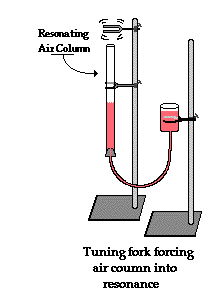hollow cylindrical tube) partially filled with water and forced into vibration by a tuning fork. The tuning fork is the object that forced the air inside of the resonance tube into resonance. As the tines of the tuning fork vibrate at their own natural frequency, they created sound waves that impinge upon the opening of the resonance tube. These impinging sound waves produced by the tuning fork force air inside of the resonance tube to vibrate at the same frequency. Yet, in the absence of resonance, the sound of these vibrations is not loud enough to discern. Resonance only occurs when the first object is vibrating at the natural frequency of the second object. So if the frequency at which the tuning fork vibrates is not identical to one of the natural frequencies of the air colu

mn inside the resonance tube, resonance will not occur and the two objects will not sound out together with a loud sound. But the location of the water level can be altered by raising and lowering a reservoir of water, thus decreasing or increasing the length of the air column. As we have learned earlier, an increase in the length of a vibrational system (here, the air in the tube) increases the wavelength and decreases the natural frequency of that system. Conversely, a decrease in the length of a vibrational system decreases the wavelength and increases the natural frequency. So by raising and lowering the water level, the natural frequency of the air in the tube could be matched to the frequency at which the tuning fork vibrates. When the match is achieved, the tuning fork forces the air column inside of the resonance tube to vibrate at its own natural frequency and resonance is achieved. The result of resonance is always a big vibration – that is, a loud sound.

Polarization Of Waves

A light wave is an electromagnetic wave that travels through the vacuum of outer space. Light waves are produced by vibrating electric charges.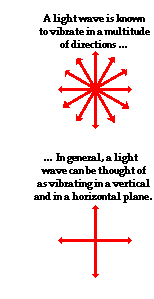Let’s suppose that we use the customary slinky to model the behavior of an electromagnetic wave. As an electromagnetic wave traveled towards you, then you would observe the vibrations of the slinky occurring in more than one plane of vibration. This is quite different than what you might notice if you were to look along a slinky and observe a slinky wave traveling towards you. Indeed, the coils of the slinky would be vibrating back and forth as the slinky approached; yet these vibrations would occur in a single plane of ispace. That is, the coils of the slinky might vibrate up and down or left and right. Yet regardless of their direction of vibration, they would be moving along the same linear direction as you sighted along the slinky. If a slinky wave were an electromagnetic wave, then the vibrations of the slinky would occur in multiple planes. Unlike a usual slinky wave, the electric and magnetic vibrations of an electromagnetic wave occur in numerous planes. A light wave that is vibrating in more than one plane is referred to as unpolarized light. Light emitted by the sun, by a lamp in the classroom, or by a candle flame is unpolarized light. Such light waves are created by electric charges that vibrate in a variety of directions, thus creating an electromagnetic wave that vibrates in a variety of directions. This concept of unpolarized light is rather difficult to visualize. In general, it is helpful to picture unpolarized light as a wave that has an average of half its vibrations in a horizontal plane and half of its vibrations in a vertical plane.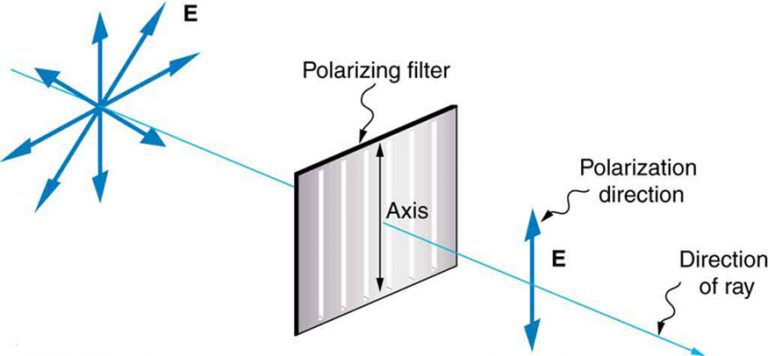Polarization by Use of a Polaroid Filter

The most common method of polarization involves the use of a Polaroid filter. Polaroid filters are made of a special material that is capable of blocking one of the two planes of vibration of an electromagnetic wave. (Remember, the notion of two planes or directions of vibration is merely a simplification that helps us to visualize the wavelike nature of the electromagnetic wave.) In this sense, a Polaroid serves as a device that filters out one-half of the vibrations upon transmission of the light through the filter. When unpolarized light is transmitted through a Polaroid filter, it emerges with one-half the intensity and with vibrations in a single plane; it emerges as polarized light.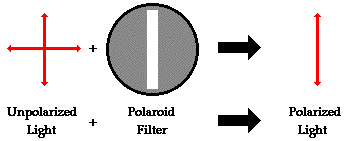A Polaroid filter is able to polarize light because of the chemical composition of the filter material. The filter can be thought of as having long-chain molecules that are aligned within the filter in the same direction. During the fabrication of the filter, the long-chain molecules are stretched across the filter so that each molecule is (as much as possible) aligned in say the vertical direction. As unpolarized light strikes the filter, the portion of the waves vibrating in the vertical direction are absorbed by the filter. The general rule is that the electromagnetic vibrations that are in a direction parallel to the alignment of the molecules are absorbed.

The alignment of these molecules gives the filter a polarization axis. This polarization axis extends across the length of the filter and only allows vibrations of the electromagnetic wave that are parallel to the axis to pass through. Any vibrations that are perpendicular to the polarization axis are blocked by the filter. Thus, a Polaroid filter with its long-chain molecules aligned horizontally will have a polarization axis aligned vertically. Such a filter will block all horizontal vibrations and allow the vertical vibrations to be transmitted (see diagram above). On the other hand, a Polaroid filter with its long-chain molecules aligned vertically will have a polarization axis aligned horizontally; this filter will block all vertical vibrations and allow the horizontal vibrations to be transmitted.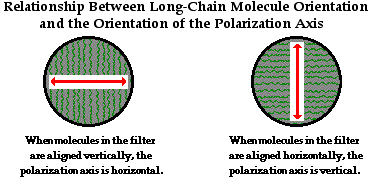Polarization of light by use of a Polaroid filter is often demonstrated in a Physics class through a variety of demonstrations. Filters are used to look through and view objects. The filter does not distort the shape or dimensions of the object; it merely serves to produce a dimmer image of the object since one-half of the light is blocked as it passed through the filter. A pair of filters is often placed back to back in order to view objects looking through two filters. By slowly rotating the second filter, an orientation can be found in which all the light from an object is blocked and the object can no longer be seen when viewed through two filters. What happened? In this demonstration, the light was polarized upon passage through the first filter; perhaps only vertical vibrations were able to pass through. These vertical vibrations were then blocked by the second filter since its polarization filter is aligned in a horizontal direction. While you are unable to see the axes on the filter, you will know when the axes are aligned perpendicular to each other because with this orientation, all light is blocked. So by use of two filters, one can completely block all of the light that is incident upon the set; this will only occur if the polarization axes are rotated such that they are perpendicular to each other.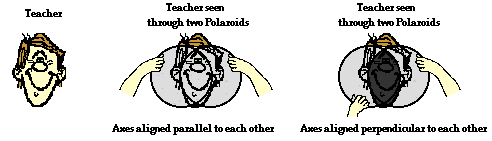A picket-fence analogy is often used to explain how this dual-filter demonstration works. A picket fence can act as a polarizer by transforming an unpolarized wave in a rope into a wave that vibrates in a single plane. The spaces between the pickets of the fence will allow vibrations that are parallel to the spacings to pass through while blocking any vibrations that are perpendicular to the spacings. Obviously, a vertical vibration would not have the room to make it through a horizontal spacing. If two picket fences are oriented such that the pickets are both aligned vertically, then vertical vibrations will pass through both fences. On the other hand, if the pickets of the second fence are aligned horizontally, then the vertical vibrations that pass through the first fence will be blocked by the second fence. This is depicted in the diagram below.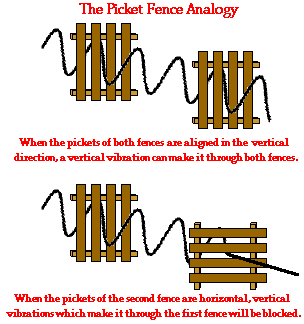In the same manner, two Polaroid filters oriented with their polarization axes perpendicular to each other will block all the light. Now that’s a pretty cool observation that could never be explained by a particle view of light.

### Polarization by Reflection

Unpolarized light can also undergo polarization by reflection off of nonmetallic surfaces. The extent to which polarization occurs is dependent upon the angle at which the light approaches the surface and upon the material that the surface is made of. Metallic surfaces reflect light with a variety of vibrational directions; such reflected light is unpolarized. However, nonmetallic surfaces such as asphalt roadways, snowfields and water reflect light such that there is a large concentration of vibrations in a plane parallel to the reflecting surface. A person viewing objects by means of light reflected off of nonmetallic surfaces will often perceive a glare if the extent of polarization is large. Fishermen are familiar with this glare since it prevents them from seeing fish that lie below the water. Light reflected off a lake is partially polarized in a direction parallel to the water’s surface. Fishermen know that the use of glare-reducing sunglasses with the proper polarization axis allows for the blocking of this partially polarized light. By blocking the plane-polarized light, the glare is reduced and the fisherman can more easily see fish located under the water.### Polarization by Refraction

Polarization can also occur by the refraction of light. Refraction occurs when a beam of light passes from one material into another material. At the surface of the two materials, the path of the beam changes its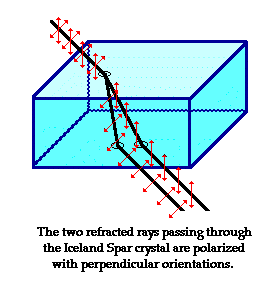direction. The refracted beam acquires some degree of polarization. Most often, the polarization occurs in a plane perpendicular to the surface. The polarization of refracted light is often demonstrated in a Physics class using a unique crystal that serves as a double-refracting crystal. Iceland Spar, a rather rare form of the mineral calcite, refracts incident light into two different paths. The light is split into two beams upon entering the crystal. Subsequently, if an object is viewed by looking through an Iceland Spar crystal, two images will be seen. The two images are the result of the double refraction of light. Both refracted light beams are polarized – one in a direction parallel to the surface and the other in a direction perpendicular to the surface. Since these two refracted rays are polarized with a perpendicular orientation, a polarizing filter can be used to completely block one of the images. If the polarization axis of the filter is aligned perpendicular to the plane of polarized light, the light is completely blocked by the filter; meanwhile the second image is as bright as can be. And if the filter is then turned 90-degrees in either direction, the second image reappears and the first image disappears. Now that’s pretty neat observation that could never be observed if light did not exhibit any wavelike behavior.

## STEM Elearning

We at FAWE have built this platform to aid learners, trainers and mentors get practical help with content, an interactive platform and tools to power their teaching and learning of STEM subjects, more